# Methods and formulas for 1-Sample Sign

Select the method of your choice.

## P-value for the exact method

Minitab uses the binomial distribution to calculate the p-value for samples up to size 50 (n ≤ 50). For a sample size n (after omitting any observations that are equal to the hypothesized median value) and a probability of occurrence of p = 0.5 under the null hypothesis, the calculation of the p-value depends on the alternative hypothesis.

Alternative hypothesis P-value
H1: Median > Hypothesized median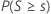H1: Median < Hypothesized median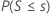H1: Median ≠ Hypothesized median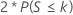### Notation

TermDescription
nthe observed number of data points after omitting any observations that are equal to the hypothesized median value
sthe observed number of data points that are greater than the hypothesized median
Sa random variable that follows a binomial distribution with n trials and a 0.5 probability of an event, B(n, 0.5)
k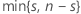## P-value for the normal approximation method

Minitab uses a normal approximation to the binomial distribution to calculate the p-value for samples that are larger than 50 (n > 50). Specifically: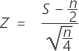is approximately distributed as a normal distribution with a mean of 0 and a standard deviation of 1, N(0,1).

where S, the number of observations that are above the median, has the binomial distribution with n as the number of trials and p = 0.5 as the probability of success under the null hypothesis, B(n, 0.5).

The normal approximation p-value for the three alternative hypotheses uses a continuity correction of 0.5.

Alternative hypothesis P-value
H1: Median > Hypothesized median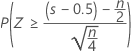H1: Median < Hypothesized median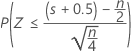H1: Median ≠ Hypothesized median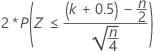### Notation

TermDescription
nthe observed number of data points after omitting any observations that are equal to the hypothesized median value
sthe observed number of data points that are greater than the hypothesized median
Sa random variable that has the binomial distribution with n as the number of trials and p = 0.5 as the probability of a success, B(n, 0.5)
k## Confidence interval

The 1-sample sign test does not always achieve the confidence level that you specify because the sign test statistic is discrete. Because of this, Minitab calculates 3 confidence intervals with varying levels of precision.

### Procedure

1. Minitab orders the observations such that X(1)< X(2)< ... < X(n), where X(i ) is the ith smallest observation.
2. For the specified confidence level (γ), the first interval is the closest exact interval with confidence ≤ γ. The third interval is the closest exact interval with confidence ≥ γ. Let d be the largest integer such that,
• P (B < d) < (1 – γ) / 2.

B has a binomial distribution with parameters sample size n and probability of occurrence p = 0.5.

3. The first interval is from X(d + 1) to X(nd) and the third interval is from X(d ) to X(nd + 1).
4. Minitab calculates the middle confidence interval by a nonlinear interpolation (NLI) procedure that was developed by Hettmansperger and Sheather1. Let γd + 1 be the confidence level of the first interval, and γd be the confidence level of the third interval.

The lower endpoint of the interpolation interval is given by:

• X(d) + λ (X(d + 1)X(d))

The upper endpoint is given by:

• X(nd + 1)λ (X(nd + 1)X(nd))
1 T.P. Hettmansperger and S.J. Sheather (1986). "Confidence Intervals Based on Interpolated Order Statistics," Statistics and Probability Letters, 4(2), 75-79.
By using this site you agree to the use of cookies for analytics and personalized content.  Read our policy